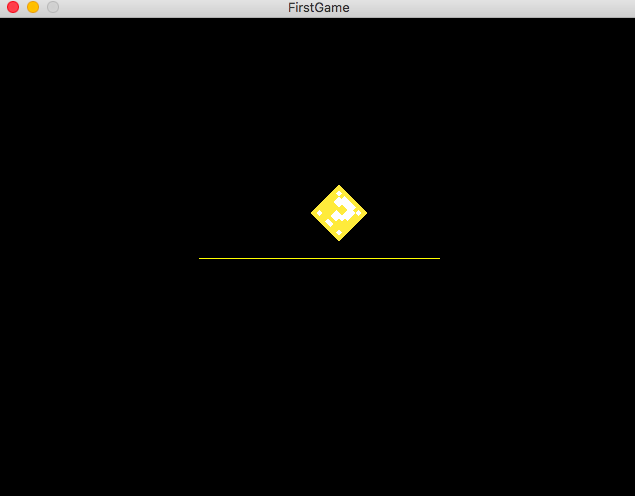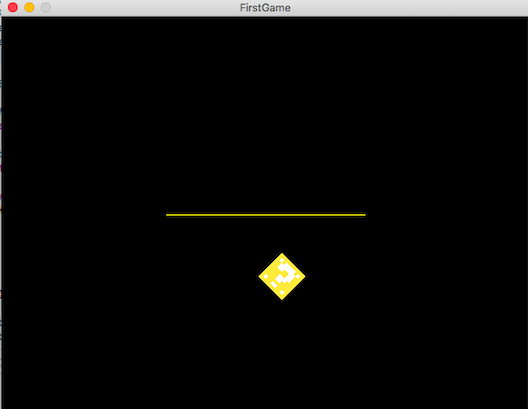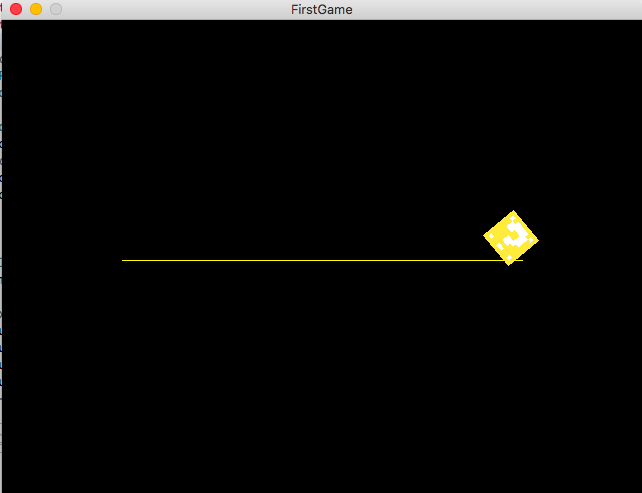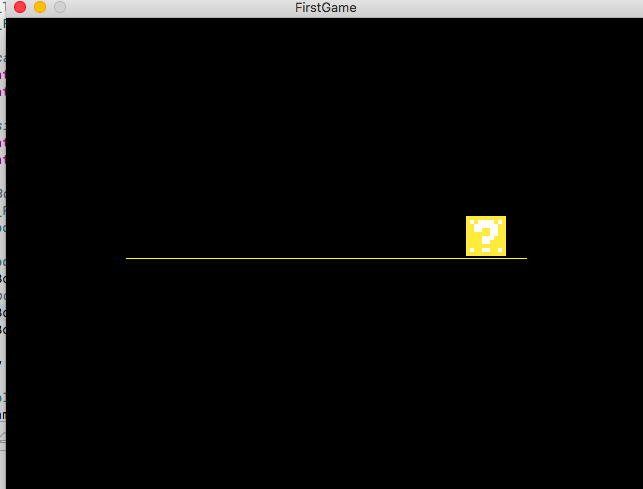# Box2D and SDL2: No Collision after Rendering

Hey guys,

Currently I am figuring out to migrate Box2D to an SDL2 project. There are several problems I cam across an solved:

• changing the “pixel coordinates” to cartesian coordinates and
• setting a scale factor (Pixels to Meters)
• drawing the whole thing

And it actually works, but for some reason the collision does not work anymore. I tried the Box2D Code in the testbed and there it works. That means the failure has to be located in SDL - in the rendering or in the translating from MKS to Pixels. So I thought you guys could help me out.

Normally it should look like this (Testbed):

But in SDL, it just “falls through” and doesn’t collide at all (ignore the low framerate, my laptop is just to weak to record something):

Here is my source code:

``````#include <iomanip>
#include <Box2D/Box2D.h>
#include <iostream>
#include <SDL2/SDL.h>

b2World* world;

const int MET2PIX = 80; // 640 / 80 = 8

const int WIDTH = 640;
const int HEIGHT = 480;

const int SCALED_WIDTH = WIDTH / MET2PIX; // 4 | 3
const int SCALED_HEIGHT = HEIGHT / MET2PIX;

using namespace std;

int main()
{
SDL_Init(SDL_INIT_EVERYTHING);

SDL_Window *window = SDL_CreateWindow("FirstGame", SDL_WINDOWPOS_CENTERED,
SDL_WINDOWPOS_CENTERED, WIDTH, HEIGHT, SDL_WINDOW_SHOWN);

SDL_Renderer *renderer = SDL_CreateRenderer(window, -1, SDL_RENDERER_ACCELERATED | SDL_RENDERER_PRESENTVSYNC);

world = new b2World(b2Vec2(0.0f, 9.81f));

// LineGround
b2BodyDef myGroundDef;
myGroundDef.type = b2_staticBody;
myGroundDef.position.Set(0, 0); // set the starting position x and y cartesian
myGroundDef.angle = 0;

b2Body* groundLineBody = world->CreateBody(&myGroundDef);

b2EdgeShape edgeShape;
edgeShape.Set( b2Vec2(-1.5,0), b2Vec2(1.5,0) ); // length -> coordinate vector from to vector

b2FixtureDef edgeFixtureDef;
edgeFixtureDef.shape = &edgeShape;
groundLineBody->CreateFixture(&edgeFixtureDef);

// Box
SDL_Rect box;
b2Body* Body;

b2BodyDef boxBodyDef;
boxBodyDef.type = b2_dynamicBody;
boxBodyDef.angle = 45;
boxBodyDef.position.Set(0, 2.5);

Body = world->CreateBody(&boxBodyDef);

b2PolygonShape dynamicBox;
dynamicBox.SetAsBox(0.25, 0.25); // will be 0.5 x 0.5

b2FixtureDef fixtureDef;
fixtureDef.shape = &dynamicBox;
fixtureDef.density = 1;
fixtureDef.friction = 0.3f;
fixtureDef.restitution = 0.5f;
Body->CreateFixture(&fixtureDef);

// box: convert Metres back to Pixels for width and height
box.w = 0.25 * MET2PIX;
box.h = 0.25 * MET2PIX;

bool close_game = false;
SDL_Event event;

// The game Loop
while(close_game != true)
{
b2Vec2 pos = Body->GetPosition(); // Body = Body from box

while(SDL_PollEvent(&event))
{
if(event.type == SDL_QUIT)
close_game = true;

else if(event.key.keysym.sym == SDLK_ESCAPE)
close_game = true;
}

box.x = ((SCALED_WIDTH / 2.0f) + pos.x) * MET2PIX;
box.y = (-(SCALED_HEIGHT / 2.0f) + pos.y) * MET2PIX;

cout << "X of box:" << setprecision(20) << box.x << endl;
cout << "Y of box:" << setprecision(20) << box.y << endl;

SDL_RenderClear(renderer);
SDL_SetRenderDrawColor(renderer, 255, 255, 0, 0);

// Draw ground platform
SDL_SetRenderDrawColor(renderer, 255, 255, 0, 0);
SDL_RenderDrawLine(renderer, ((SCALED_WIDTH / 2.0f) + edgeShape.m_vertex1.x) * MET2PIX, ((SCALED_HEIGHT / 2.0f) + edgeShape.m_vertex1.y) * MET2PIX, ((SCALED_WIDTH / 2.0f) + edgeShape.m_vertex2.x) * MET2PIX, ((SCALED_HEIGHT / 2.0f) + edgeShape.m_vertex2.y) * MET2PIX);

// Draw our Box
SDL_RenderDrawRect(renderer, &box);
SDL_RenderFillRect(renderer, &box);

SDL_SetRenderDrawColor(renderer, 0, 0, 0, 0);
SDL_RenderPresent(renderer);

world->Step(1.0f / 60.0f, 6.0f, 2.0f); // update

}

delete world;

SDL_DestroyRenderer(renderer);
SDL_DestroyWindow(window);
SDL_Quit();
return EXIT_SUCCESS;
}
``````

I appreciate any support!
eder

There are some things I don’t understand:

1. Your box dimensions are 0.5 x 0.5 metres, but when you convert these to pixels you are setting box.w and box.h to `0.25 * MET2PIX` (not `0.5 * MET2PIX`). Why?

2. You are always drawing your box axis aligned, apparently ignoring its angle.

3. Body->GetPosition() returns the center coordinates, I think, but you seem to be converting them to the corner coordinates when rendering.

If you cannot trust that the graphics being rendered by SDL accurately correspond with the BOX2D coordinates it is difficult to analyze why the collision seems to be failing.

Thank you @rtrussell for your respond!
I fixed 1 and 2 and added a short Box Texture.

``````#include <iomanip>
#include <Box2D/Box2D.h>
#include <iostream>
#include <SDL2/SDL.h>
#include <SDL2_image/SDL_image.h>

b2World* world;

const int MET2PIX = 80; // 640 / 80 = 8

const int WIDTH = 640;
const int HEIGHT = 480;

const int SCALED_WIDTH = WIDTH / MET2PIX; // 4 | 3
const int SCALED_HEIGHT = HEIGHT / MET2PIX;

using namespace std;

int main()
{
SDL_Init(SDL_INIT_EVERYTHING);

SDL_Window *window = SDL_CreateWindow("FirstGame", SDL_WINDOWPOS_CENTERED,
SDL_WINDOWPOS_CENTERED, WIDTH, HEIGHT, SDL_WINDOW_SHOWN);

SDL_Renderer *renderer = SDL_CreateRenderer(window, -1, SDL_RENDERER_ACCELERATED | SDL_RENDERER_PRESENTVSYNC);

world = new b2World(b2Vec2(0.0f, 9.81f));

// cartesian origin
float ground_x = 0.0f;
float ground_y = 0.0f;

// start ground point
b2Vec2 startpoint;
startpoint.x = -1.5f;
startpoint.y = 0;

// end ground point
b2Vec2 endpoint;
endpoint.x = 1.5;
endpoint.y = 0;

// LineGround
b2BodyDef myGroundDef;
myGroundDef.type = b2_staticBody;
myGroundDef.position.Set(ground_x, ground_y); // set the starting position x and y cartesian
myGroundDef.angle = 0;

b2Body* groundLineBody = world->CreateBody(&myGroundDef);

b2EdgeShape edgeShape;
edgeShape.Set( startpoint, endpoint ); // length -> coordinate vector from to vector

b2FixtureDef edgeFixtureDef;
edgeFixtureDef.shape = &edgeShape;
groundLineBody->CreateFixture(&edgeFixtureDef);

SDL_Surface* tmp_sprites;
if(!tmp_sprites)
return EXIT_FAILURE;

SDL_Texture* texture_box = SDL_CreateTextureFromSurface(renderer, tmp_sprites);
SDL_FreeSurface(tmp_sprites);

// cartesian origin box
float x_box = 0;
float y_box= 2.5f;

// size of box
float w_box = 0.5;
float h_box = 0.5;

// Box
SDL_Rect box;
b2Body* Body;

b2BodyDef boxBodyDef;
boxBodyDef.type = b2_dynamicBody;
boxBodyDef.angle = 45;
boxBodyDef.position.Set(x_box, y_box);

Body = world->CreateBody(&boxBodyDef);

b2PolygonShape dynamicBox;
dynamicBox.SetAsBox(w_box / 2.0f, h_box / 2.0f); // will be 0.5 x 0.5

b2FixtureDef fixtureDef;
fixtureDef.shape = &dynamicBox;
fixtureDef.density = 1;
fixtureDef.friction = 0.3f;
fixtureDef.restitution = 0.5f;
Body->CreateFixture(&fixtureDef);

// box: convert Metres back to Pixels for width and height
box.w = w_box * MET2PIX;
box.h = h_box * MET2PIX;

// point to center of box for angle
SDL_Point center;
center.x = box.w * 0.5f;
center.y = box.h - (box.w * 0.5);

bool close_game = false;
SDL_Event event;

// The game Loop
while(close_game != true)
{
b2Vec2 pos = Body->GetPosition(); // Body = Body from box
float angle = Body->GetAngle();

while(SDL_PollEvent(&event))
{
if(event.type == SDL_QUIT)
close_game = true;

else if(event.key.keysym.sym == SDLK_ESCAPE)
close_game = true;
}

box.x = ((SCALED_WIDTH / 2.0f) + pos.x) * MET2PIX;
box.y = (-(SCALED_HEIGHT / 2.0f) + pos.y) * MET2PIX;

cout << "X of box:" << setprecision(20) << box.x << endl;
cout << "Y of box:" << setprecision(20) << box.y << endl;

SDL_RenderClear(renderer);
SDL_SetRenderDrawColor(renderer, 255, 255, 0, 0);

// Draw ground platform
SDL_SetRenderDrawColor(renderer, 255, 255, 0, 0);
SDL_RenderDrawLine(renderer, ((SCALED_WIDTH / 2.0f) + edgeShape.m_vertex1.x) * MET2PIX, ((SCALED_HEIGHT / 2.0f) + edgeShape.m_vertex1.y) * MET2PIX, ((SCALED_WIDTH / 2.0f) + edgeShape.m_vertex2.x) * MET2PIX, ((SCALED_HEIGHT / 2.0f) + edgeShape.m_vertex2.y) * MET2PIX);

// Draw our Box
SDL_RenderCopyEx(renderer, texture_box, NULL, &box, angle, &center, SDL_FLIP_NONE);

SDL_SetRenderDrawColor(renderer, 0, 0, 0, 0);
SDL_RenderPresent(renderer);

world->Step(1.0f / 60.0f, 6.0f, 2.0f); // update

}

delete world;

SDL_DestroyRenderer(renderer);
SDL_DestroyWindow(window);
SDL_Quit();
return EXIT_SUCCESS;
}
``````

What do you mean by 3? How could I implement that so that it works?
It still does not collide.Strange enough I found an example online (but that takes pixels instead of MKS, which runs poorly - but this collides. The only difference is that the example uses a groundBox with SDL_Rect instead if just a Line (SDL_DrawLine). I tried changing my code like this but it didn’t make a difference.
This is the example:

``````int gameLoop(SDL_Window *window, SDL_Renderer *renderer)
{
SDL_Surface* tmp_sprites;
if(!tmp_sprites)
return FAILED_TO_CREATE_TEXTURE;

SDL_Texture* texture_box = SDL_CreateTextureFromSurface(renderer, tmp_sprites);
SDL_FreeSurface(tmp_sprites);

// initialization
bool close_game = false;
SDL_Event event;

// parameters of the platform
float x_pos_rect = -2.0f;
float y_pos_rect = 219.9f;
float width_rect = 320;
float height_rect = 5;

// parameters of the box itself
float x_pos_box = 0;// * P2M;
float y_pos_box = 20.0f;// * P2M;
float width_box = 10;// * P2M;
float height_box = 10;// * P2M;

SDL_Rect ground;
ground.x = x_pos_rect;
ground.y = y_pos_rect;
ground.w = width_rect;
ground.h = height_rect;

SDL_Rect box;

box.x = x_pos_box;
box.y = y_pos_box;
box.w = width_box;
box.h = height_box;

world = new b2World(b2Vec2(0.0f, 100.81f)); // the higher, the more erdanziehungskraft is set
world->SetAllowSleeping(false); // sets sleeping of objects if they havent been touched recently to false

b2Body *groundBody;

b2BodyDef groundBodyDef;
groundBodyDef.type = b2_staticBody;
groundBodyDef.position.Set(x_pos_rect, y_pos_rect); // here
groundBodyDef.angle = 0;
groundBody = world->CreateBody(&groundBodyDef);

b2PolygonShape groundBox;
groundBox.SetAsBox(width_rect, height_box);

b2FixtureDef boxShapeDef;
boxShapeDef.shape = &groundBox; // a rectangle in this case
boxShapeDef.density = 0.0f; // how heavy it is in relation to its area
groundBody->CreateFixture(&groundBox, 0);

b2Body* Body;

b2BodyDef ballBodyDef;
ballBodyDef.type = b2_dynamicBody;
ballBodyDef.angle = 0.0f;
ballBodyDef.position.Set(200, 40); // set the position of the box where it should start falling *P2M on both if pixels to meter

Body = world->CreateBody(&ballBodyDef); // ballBodyDef informations above are now saved in *Body*

b2PolygonShape dynamicBox;
dynamicBox.SetAsBox(width_box / 2, height_box / 2); // Pixel to meter, SetAsBox always gets "the half"

b2FixtureDef fixtureDef;
fixtureDef.shape = &dynamicBox; // also a rectangle in this case
fixtureDef.density = 1; // how heavy it is in relation to its area The area of the fixture is multiplied by the density of the fixture to calculate its mass, and this becomes the mass of the body By now you are probably wondering why these bodies don't spin around as expected --> density
fixtureDef.friction = 0.3f; // how slippery it is
fixtureDef.restitution = 0.5f; // how bouncy the object is - 0 = it wont jump up anymore
Body->CreateFixture(&fixtureDef); // take the Body properties and make a fixture

// The game Loop
while(close_game != true)
{
while(SDL_PollEvent(&event))
{
if(event.type == SDL_QUIT)
close_game = true;

else if(event.key.keysym.sym == SDLK_ESCAPE)
close_game = true;
}

b2Vec2 pos = Body->GetPosition(); // Body = Body from box
float angle = Body->GetAngle();

box.x = pos.x;
box.y = pos.y;

cout << "X of box:" << setprecision(20) << pos.x << endl;
cout << "Y of box:" << setprecision(20) << pos.y << endl;
cout << "angle of box: " << setprecision(20) << angle << endl;

SDL_RenderClear(renderer);
SDL_SetRenderDrawColor(renderer, 255, 255, 0, 0);

// Draws our little box                             rotate
//                                                    |
SDL_RenderCopyEx(renderer, texture_box, NULL, &box, angle, NULL, SDL_FLIP_NONE);

// Draws ground platform
SDL_SetRenderDrawColor(renderer, 255, 255, 0, 0);
SDL_RenderFillRect(renderer, &ground);

SDL_SetRenderDrawColor(renderer, 0, 0, 0, 0);
SDL_RenderPresent(renderer);

world->Step(1.0f / 60.0f, 6.0f, 2.0f); // update

}

delete world;

return EXIT_SUCCESS;
}
``````

I just don’t get it why here colliding works and mine won’t.

When calling `SDL_RenderCopyEx()` I think you should set the center parameter to NULL (so that it rotates around the center of the box) and offset the box.x and box.y parameters so that the center is in the correct place, something like this:

``````    box.x = ((SCALED_WIDTH / 2.0f) + pos.x) * MET2PIX - box.w / 2;
box.y = (-(SCALED_HEIGHT / 2.0f) + pos.y) * MET2PIX - box.h / 2;
``````

I also don’t understand why there is a minus sign at the start of the y term; if the Box2D coordinate origin is in the center of the screen shouldn’t it be:

``````    box.x = ((SCALED_WIDTH / 2.0f) + pos.x) * MET2PIX - box.w / 2;
box.y = ((SCALED_HEIGHT / 2.0f) + pos.y) * MET2PIX - box.h / 2;
``````

With the position initialised to:

``````float y_box = -2.5f;
``````

If you are drawing the box with a large y-offset it would explain why it is seeming not to collide!

Thank you, I finally get a collision!
I think I made some mathematical mistakes when I calculated everything for the renderer.

Just one last problem, when it finishes colliding the box sits like this:Normally it should “correct itself” (like in real physics) and the box should after colliding “stay straight” like in real life - why isn’t it the case here (again, in the testbed everything works)? So in the end, after it collided and “corrected itself” it should sit like this:I guess I have to add something to the renderering-process, so I fired up Google to see how I can “rotate” an SDL_Rect but I stepped upon this post where it says it is basically not possible to achive what I want. Now I am confused. Is this even possible? And if yes - how? I read through the wiki but didn’t find a corresponding function from the API to achieve this.

The angle returned from `Body->GetAngle()` is in radians but the angle required by `SDL_RenderCopyEx()` is in degrees, so you need to multiply by 180 / PI which you are not doing. You will also need to reverse the sign of the angle: clockwise angles are negative in Box2D but positive in SDL.

You are currently rotating the texture which represents the box, which should be all you need to do. So long as you rotate it by the correct angle in the correct direction all should be well.

Finally, with the help of @rtrussell it works now! Thank you so much!

Here my full source code, maybe it helps someone too:

``````#include <iomanip>
#include <Box2D/Box2D.h>
#include <iostream>
#include <cmath>
#include <SDL2/SDL.h>
#include <SDL2_image/SDL_image.h>

b2World* world;

const int MET2PIX = 80; // 640 / 80 = 8

const int WIDTH = 640;
const int HEIGHT = 480;

const int SCALED_WIDTH = WIDTH / MET2PIX; // 4 | 3
const int SCALED_HEIGHT = HEIGHT / MET2PIX;

//1 rad × 180/π = 57,296°
const float RAD2DEG = 180 / M_PI;

using namespace std;

int main()
{
SDL_Init(SDL_INIT_EVERYTHING);

SDL_Window *window = SDL_CreateWindow("FirstGame", SDL_WINDOWPOS_CENTERED,
SDL_WINDOWPOS_CENTERED, WIDTH, HEIGHT, SDL_WINDOW_SHOWN);

SDL_Renderer *renderer = SDL_CreateRenderer(window, -1, SDL_RENDERER_ACCELERATED | SDL_RENDERER_PRESENTVSYNC);

world = new b2World(b2Vec2(0.0f, 9.81f));

// cartesian origin
float ground_x = 0.0f;
float ground_y = 0.0f;

// start ground point
b2Vec2 startpoint;
startpoint.x = -2.5f;
startpoint.y = 0;

// end ground point
b2Vec2 endpoint;
endpoint.x = 2.5;
endpoint.y = 0;

// LineGround
b2BodyDef myGroundDef;
myGroundDef.type = b2_staticBody;
myGroundDef.position.Set(ground_x, ground_y); // set the starting position x and y cartesian
myGroundDef.angle = 0;

b2Body* groundLineBody = world->CreateBody(&myGroundDef);

b2EdgeShape edgeShape;
edgeShape.Set( startpoint, endpoint ); // length -> coordinate vector from to vector

b2FixtureDef edgeFixtureDef;
edgeFixtureDef.shape = &edgeShape;
groundLineBody->CreateFixture(&edgeFixtureDef);

SDL_Surface* tmp_sprites;
if(!tmp_sprites)
return EXIT_FAILURE;

SDL_Texture* texture_box = SDL_CreateTextureFromSurface(renderer, tmp_sprites);
SDL_FreeSurface(tmp_sprites);

// cartesian origin box
float x_box = 0;
float y_box = -2.5f;

// size of box
float w_box = 0.5;
float h_box = 0.5;

// Box
SDL_Rect box;
b2Body* Body;

b2BodyDef boxBodyDef;
boxBodyDef.type = b2_dynamicBody;
boxBodyDef.angle = 45; // flips the whole thing -> 180 grad drehung
//boxBodyDef.angle = 0;
boxBodyDef.position.Set(x_box, y_box);

Body = world->CreateBody(&boxBodyDef);

b2PolygonShape dynamicBox;
dynamicBox.SetAsBox(w_box / 2.0f, h_box / 2.0f); // will be 0.5 x 0.5

b2FixtureDef fixtureDef;
fixtureDef.shape = &dynamicBox;
fixtureDef.density = 1;
fixtureDef.friction = 0.3f;
fixtureDef.restitution = 0.5f;
Body->CreateFixture(&fixtureDef);

// box: convert Metres back to Pixels for width and height
box.w = w_box * MET2PIX;
box.h = h_box * MET2PIX;

bool close_game = false;
SDL_Event event;

// The game Loop
while(close_game != true)
{
b2Vec2 pos = Body->GetPosition(); // Body = Body from box
float angle = Body->GetAngle();

while(SDL_PollEvent(&event))
{
if(event.type == SDL_QUIT)
close_game = true;

else if(event.key.keysym.sym == SDLK_ESCAPE)
close_game = true;
}

box.x = ((SCALED_WIDTH / 2.0f) + pos.x) * MET2PIX - box.w / 2;
box.y = ((SCALED_HEIGHT / 2.0f) + pos.y) * MET2PIX - box.h / 2;

cout << "X of box:" << setprecision(20) << box.x << endl;
cout << "Y of box:" << setprecision(20) << box.y << endl;

SDL_RenderClear(renderer);
SDL_SetRenderDrawColor(renderer, 255, 255, 0, 0);

// Draw ground platform
SDL_SetRenderDrawColor(renderer, 255, 255, 0, 0);
SDL_RenderDrawLine(renderer, ((SCALED_WIDTH / 2.0f) + edgeShape.m_vertex1.x) * MET2PIX, ((SCALED_HEIGHT / 2.0f) + edgeShape.m_vertex1.y) * MET2PIX, ((SCALED_WIDTH / 2.0f) + edgeShape.m_vertex2.x) * MET2PIX, ((SCALED_HEIGHT / 2.0f) + edgeShape.m_vertex2.y) * MET2PIX);

// Draw our Box angle 45
// Body->SetFixedRotation(true); - sets no rotation at all
SDL_RenderCopyEx(renderer, texture_box, NULL, &box, angle, NULL, SDL_FLIP_NONE);

// Draw box angle 45
//Body->SetAngularVelocity(10.0f);
//Body->SetFixedRotation(true);
//SDL_RenderDrawRect(renderer, &box);
//SDL_RenderFillRect(renderer, &box);

SDL_SetRenderDrawColor(renderer, 0, 0, 0, 0);
SDL_RenderPresent(renderer);

world->Step(1.0f / 60.0f, 6.0f, 2.0f); // update

}

delete world;

SDL_DestroyRenderer(renderer);
SDL_DestroyWindow(window);
SDL_Quit();
return EXIT_SUCCESS;
}
``````

Have a nice day!

1 Like